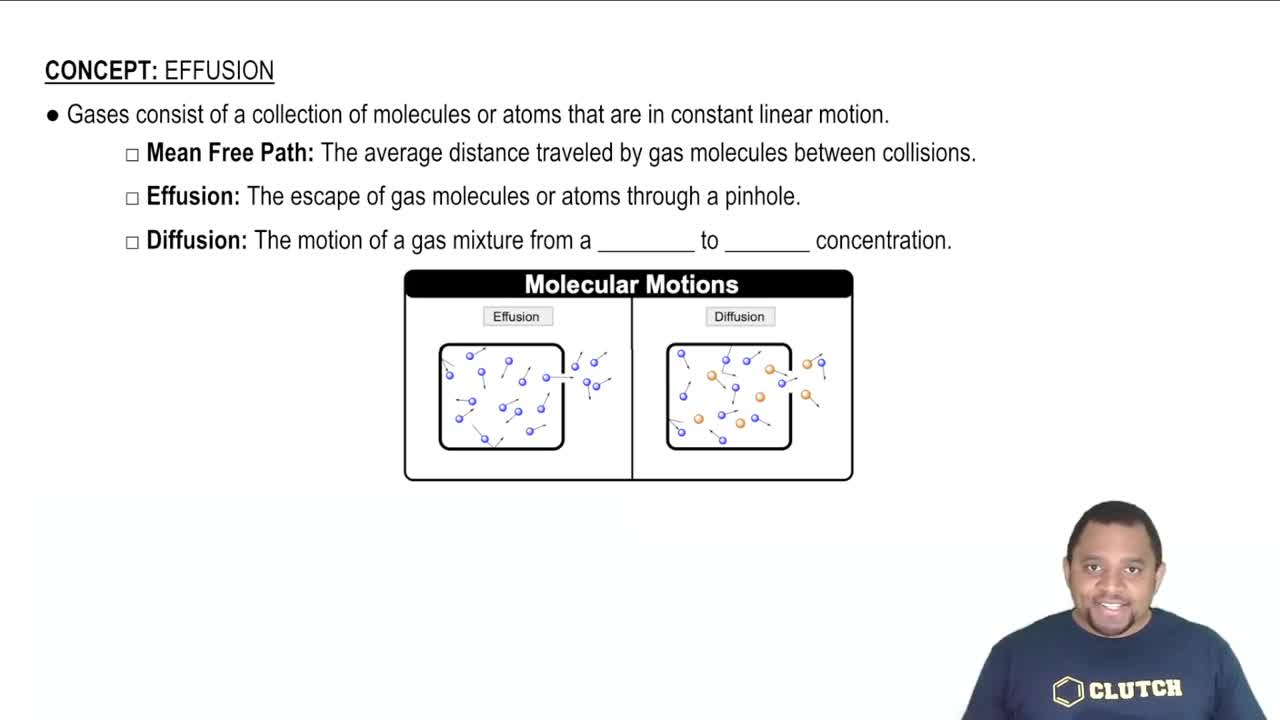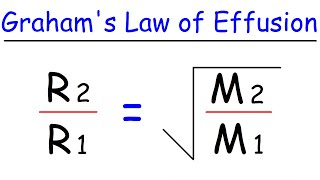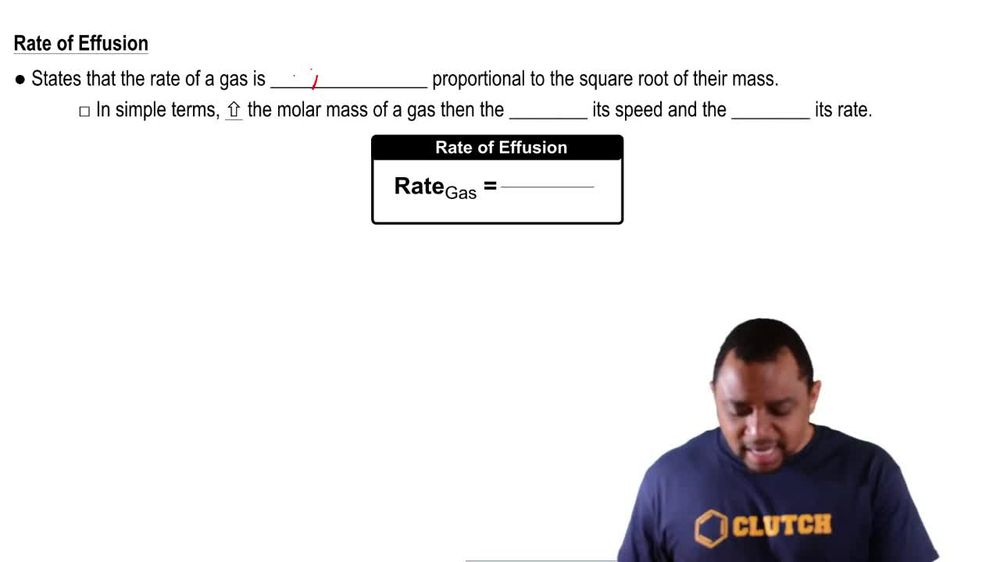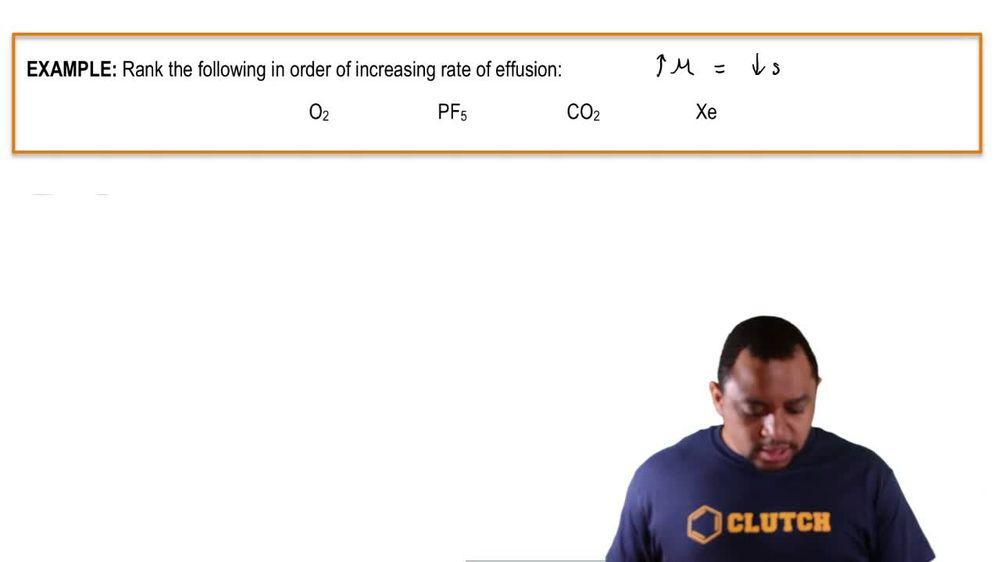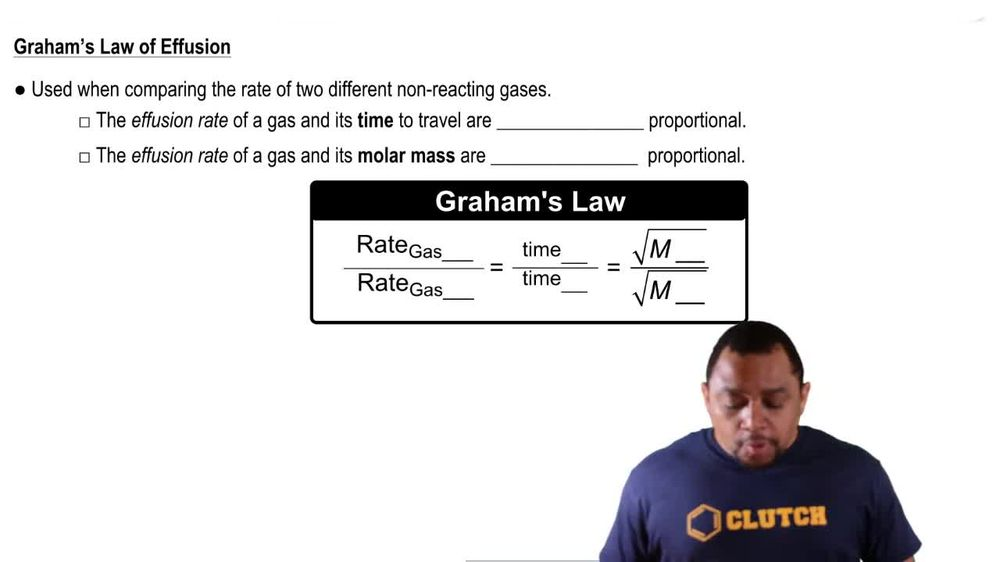Start typing, then use the up and down arrows to select an option from the list.
1. 7. Gases2. Effusion# Effusion Example 1

by Jules Bruno
123 views
1
1
here, it says to rank the following in order of increasing rate of a fusion. Remember, we said that the higher the Mueller Mass than the lower the speed or rate of a gas. So here we take a look. Oates, who has to Oxygen's The combined mass would be 32 g per mole. P F five has one phosphorus and five florins. When you add them all up together, it gives you approximately 125.97 g per mole. Carbon dioxide weighs approximately 44.1 g per mole. When you add up with one carbon and the two oxygen's and then Zen in, if you look on the periodic table, it has a wait of approximately 131.29 g per mole. We want to do it in terms of increasing rate of a fusion, which means we're going to start out with the heaviest, which has the slowest rate all the way up to the lightest one, which has the highest rate. Alright, so who has the highest smaller mass or highest weight would be Xena? Xena would be the slowest in therefore lowest rate of a fusion, then we have P F five. Then we have CO two and then, 02 ways, the least out of everyone, so it would move the fastest. So this would be our order going from the slowest rate of a fusion to be highest rate of fusion based on their individual Mueller masses.